# Basic repeated measures example

In my own personal lexicon I don't make much distinction between mixed models, repeated measures analyses, and longitudinal data analysis – the latter two are really special cases of the first, where the analysis involves experimental units measured repeatedly over time (as opposed to, say, a nested design where multiple measurements are taken, all at once, within a block). The special features of longitudinal/repeated measures analyses are:

• we often want to treat time as a continuous covariate, allowing for smooth trends (linear, quadratic, spline) over time
• we may want to account for autocorrelation in the errors at pairs of measurements depending on their distance apart in time

This is all fairly straightforward …

``````library("nlme")
library("ggplot2")
library("bbmle")
library("gridExtra")
theme_set(theme_bw())
ggplot(Orthodont, aes(x = age, y = distance, colour = Sex)) + geom_line(aes(group = Subject))
``````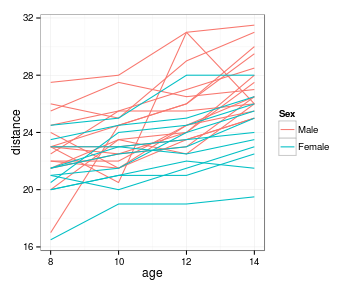``````m1 <- lme(distance ~ I(age - 11) * Sex, random = ~age | Subject, data = Orthodont)
summary(m1)
``````
``````## Linear mixed-effects model fit by REML
##  Data: Orthodont
##     AIC   BIC logLik
##   448.6 469.7 -216.3
##
## Random effects:
##  Formula: ~age | Subject
##  Structure: General positive-definite, Log-Cholesky parametrization
##             StdDev Corr
## (Intercept) 2.4055 (Intr)
## age         0.1803 -0.668
## Residual    1.3100
##
## Fixed effects: distance ~ I(age - 11) * Sex
##                        Value Std.Error DF t-value p-value
## (Intercept)           24.969    0.4860 79   51.38  0.0000
## I(age - 11)            0.784    0.0860 79    9.12  0.0000
## SexFemale             -2.321    0.7614 25   -3.05  0.0054
## I(age - 11):SexFemale -0.305    0.1347 79   -2.26  0.0264
##  Correlation:
##                       (Intr) I(g-11) SexFml
## I(age - 11)            0.102
## SexFemale             -0.638 -0.065
## I(age - 11):SexFemale -0.065 -0.638   0.102
##
## Standardized Within-Group Residuals:
##       Min        Q1       Med        Q3       Max
## -3.168078 -0.385939  0.007104  0.445155  3.849464
##
## Number of Observations: 108
## Number of Groups: 27
``````
• I think the `DF` column is actually wrong for `age` and `age:SexFemale` – these should probably be 25 as well.
• comparison of `SexFemale` only make sense (i.e. matches the conclusion we get from glancing at the plot the males have higher distance than females) if we center the `age` variable

Even though we only have 4 observations per subject, we'll try to compute the autocorrelation:

``````plot(ACF(m1), alpha = 0.05)
``````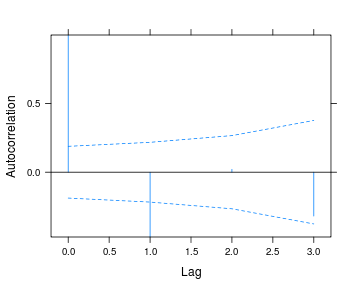There is actually sig. (negative) autocorrelation at lag-1 …

Use autoregressive-order-1 model, with order of observation in the data as a covariate

``````m2 <- update(m1, correlation = corAR1(form = ~1 | Subject))
summary(m2\$modelStruct)
``````
``````## Random effects:
##  Formula: ~age | Subject
##  Structure: General positive-definite, Log-Cholesky parametrization
##             StdDev Corr
## (Intercept) 3.0889 (Intr)
## age         0.2663 -0.831
## Residual    1.0000
##
## Correlation Structure: AR(1)
##  Formula: ~1 | Subject
##  Parameter estimate(s):
##     Phi
## -0.4733
``````

Correlation coefficient of -0.47 between successive estimates.

(It's not normally necessary to pull bits out of the fit – could just use `summary(m2)` – doing it for compactness.

If we wanted we could test the difference by AIC: adding autocorrelation seems to be justified.

``````AICtab(m1, m2)  ## slightly prettier than AIC(m1,m2)
``````
``````##    dAIC df
## m2 0.0  9
## m1 1.8  8
``````

We can plot the residuals (supposedly) corrected for the autocorrelation – but (even though the likelihood is better) the apparent autocorrelation is worse: too noisy??

``````plot(ACF(m2, resType = "normalized"), alpha = 0.05)
``````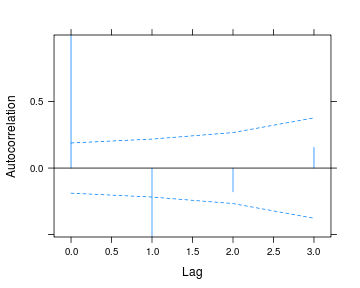Look at residuals by themselves

``````O2 <- transform(Orthodont, res = residuals(m2))
ggplot(O2, aes(x = age, y = res, colour = Sex)) + geom_line(aes(group = Subject))
``````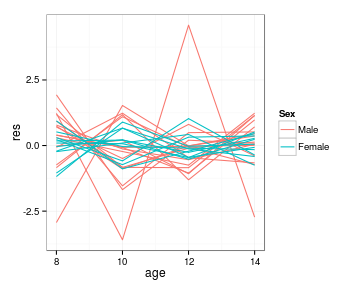Autocorrelation pattern is fairly clear from this. Also clear that one subject is a little wonky:

``````subset(O2, abs(res) > 2.5)
``````
``````##    distance age Subject  Sex    res
## 34     20.5  10     M09 Male -3.591
## 35     31.0  12     M09 Male  4.590
## 36     26.0  14     M09 Male -2.729
## 49     17.0   8     M13 Male -2.934
``````

Taking this individual out does help with normality …

``````m3 <- update(m2, subset = Subject != "M09")
library("gridExtra")
grid.arrange(qqnorm(m2), qqnorm(m3), nrow = 1)
``````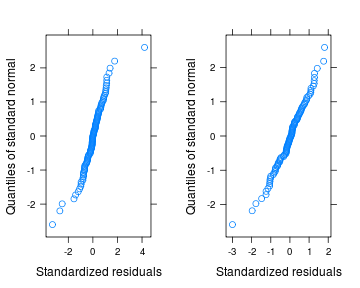Doesn't really seem to fix the ACF issue, though …

``````plot(ACF(m3, resType = "normalized"), alpha = 0.05)
``````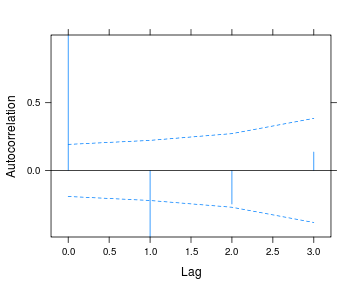## To do

• Comparison with two-stage model (use `lmList` to fit linear regressions for each individual, then analyze slope and intercept parameters?)